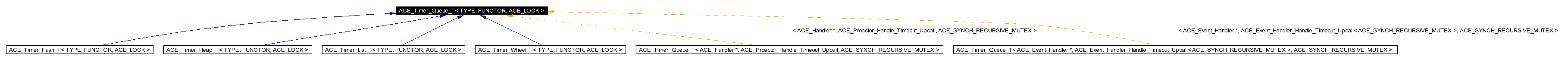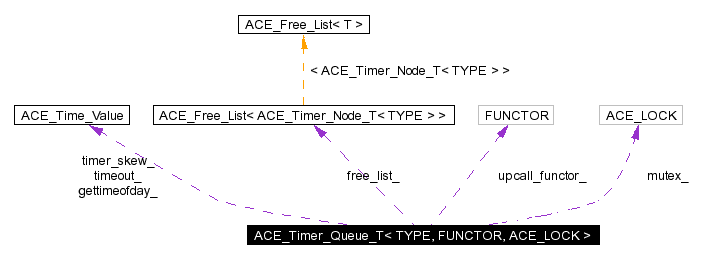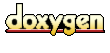Main Page   Namespace List   Class Hierarchy   Alphabetical List   Compound List   File List   Namespace Members   Compound Members   File Members   Related Pages

# ACE_Timer_Queue_T< TYPE, FUNCTOR, ACE_LOCK > Class Template Reference

Provides an interface to timers. More...

`#include <Timer_Queue_T.h>`

Inheritance diagram for ACE_Timer_Queue_T< TYPE, FUNCTOR, ACE_LOCK >:[legend]
Collaboration diagram for ACE_Timer_Queue_T< TYPE, FUNCTOR, ACE_LOCK >:[legend]
List of all members.

## Public Types

typedef ACE_Timer_Queue_Iterator_T<
TYPE, FUNCTOR, ACE_LOCK >
ITERATOR
Type of Iterator.

## Public Methods

ACE_Timer_Queue_T (FUNCTOR *upcall_functor=0, ACE_Free_List< ACE_Timer_Node_T< TYPE > > *freelist=0)
virtual ~ACE_Timer_Queue_T (void)
virtual int is_empty (void) const=0
True if queue is empty, else false.

virtual const ACE_Time_Valueearliest_time (void) const=0
virtual long schedule (const TYPE &type, const void *act, const ACE_Time_Value &future_time, const ACE_Time_Value &interval=ACE_Time_Value::zero)
virtual int reset_interval (long timer_id, const ACE_Time_Value &interval)=0
virtual int cancel (const TYPE &type, int dont_call_handle_close=1)=0
virtual int cancel (long timer_id, const void **act=0, int dont_call_handle_close=1)=0
virtual int expire (const ACE_Time_Value &current_time)
virtual int dispatch_info (const ACE_Time_Value &current_time, ACE_Timer_Node_Dispatch_Info_T< TYPE > &info)
int expire (void)
ACE_Time_Value gettimeofday (void)
void gettimeofday (ACE_Time_Value(*gettimeofday)(void))
virtual ACE_Time_Valuecalculate_timeout (ACE_Time_Value *max)
virtual ACE_Time_Valuecalculate_timeout (ACE_Time_Value *max, ACE_Time_Value *the_timeout)
void timer_skew (const ACE_Time_Value &skew)
Set the timer skew for the Timer_Queue.

const ACE_Time_Valuetimer_skew (void) const
Get the timer skew for the Timer_Queue.

ACE_LOCK & mutex (void)
Synchronization variable used by the queue.

FUNCTOR & upcall_functor (void)
Accessor to the upcall functor.

virtual ITERATORiter (void)=0
Returns a pointer to this <ACE_Timer_Queue>'s iterator.

virtual ACE_Timer_Node_T<
TYPE > *
remove_first (void)=0
Removes the earliest node from the queue and returns it.

virtual void dump (void) const
Dump the state of a object.

virtual ACE_Timer_Node_T<
TYPE > *
get_first (void)=0
Reads the earliest node from the queue and returns it.

virtual void return_node (ACE_Timer_Node_T< TYPE > *)
void preinvoke (ACE_Timer_Node_Dispatch_Info_T< TYPE > &info, const ACE_Time_Value &cur_time, const void *&upcall_act)
This method will call the preinvoke() on <functor>.

void upcall (ACE_Timer_Node_Dispatch_Info_T< TYPE > &info, const ACE_Time_Value &cur_time)
This method will call the timeout() on <functor>.

void postinvoke (ACE_Timer_Node_Dispatch_Info_T< TYPE > &info, const ACE_Time_Value &cur_time, const void *upcall_act)
This method will call the postinvoke() on <functor>.

## Protected Methods

virtual long schedule_i (const TYPE &type, const void *act, const ACE_Time_Value &future_time, const ACE_Time_Value &interval)=0
Schedule a timer.

virtual void reschedule (ACE_Timer_Node_T< TYPE > *)=0
Reschedule an "interval" <ACE_Timer_Node>.

virtual ACE_Timer_Node_T<
TYPE > *
alloc_node (void)
Factory method that allocates a new node.

virtual void free_node (ACE_Timer_Node_T< TYPE > *)
Factory method that frees a previously allocated node.

virtual int dispatch_info_i (const ACE_Time_Value &current_time, ACE_Timer_Node_Dispatch_Info_T< TYPE > &info)
Non-locking version of dispatch_info ().

## Protected Attributes

ACE_LOCK mutex_
ACE_Free_List< ACE_Timer_Node_T<
TYPE > > *
free_list_
Class that implements a free list.

ACE_Time_Value(* gettimeofday_ )(void)
Pointer to function that returns the current time of day.

FUNCTOR * upcall_functor_
Upcall functor.

int delete_upcall_functor_
To delete or not to delete is the question?

int delete_free_list_
Flag to delete only if the class created the <free_list_>.

## Private Methods

ACE_Timer_Queue_T (const ACE_Timer_Queue_T< TYPE, FUNCTOR, ACE_LOCK > &)
void operator= (const ACE_Timer_Queue_T< TYPE, FUNCTOR, ACE_LOCK > &)

## Private Attributes

ACE_Time_Value timeout_
Returned by <calculate_timeout>.

ACE_Time_Value timer_skew_
Adjusts for timer skew in various clocks.

## Detailed Description

### template<class TYPE, class FUNCTOR, class ACE_LOCK> class ACE_Timer_Queue_T< TYPE, FUNCTOR, ACE_LOCK >

Provides an interface to timers.

This is an abstract base class that provides hook for implementing specialized policies such as <ACE_Timer_List> and <ACE_Timer_Heap>.

## Member Typedef Documentation

 template typedef ACE_Timer_Queue_Iterator_T ACE_Timer_Queue_T< TYPE, FUNCTOR, ACE_LOCK >::ITERATOR
 Type of Iterator.

## Constructor & Destructor Documentation

 template ACE_Timer_Queue_T< TYPE, FUNCTOR, ACE_LOCK >::ACE_Timer_Queue_T ( FUNCTOR * upcall_functor = 0, ACE_Free_List< ACE_Timer_Node_T< TYPE > > * freelist = 0 )
 Default constructor. is the instance of the FUNCTOR to be used by the queue. If is 0, Timer Queue will create a default FUNCTOR. the freelist of timer nodes. If 0, then a default freelist will be created.

 template ACE_Timer_Queue_T< TYPE, FUNCTOR, ACE_LOCK >::~ACE_Timer_Queue_T ( void ) ` [virtual]`
 Destructor - make virtual for proper destruction of inherited classes.

 template ACE_Timer_Queue_T< TYPE, FUNCTOR, ACE_LOCK >::ACE_Timer_Queue_T ( const ACE_Timer_Queue_T< TYPE, FUNCTOR, ACE_LOCK > & ) ` [private]`

## Member Function Documentation

 template ACE_Timer_Node_T< TYPE > * ACE_Timer_Queue_T< TYPE, FUNCTOR, ACE_LOCK >::alloc_node ( void ) ` [protected, virtual]`
 Factory method that allocates a new node. Reimplemented in ACE_Timer_Heap_T< TYPE, FUNCTOR, ACE_LOCK >.

 template ACE_Time_Value * ACE_Timer_Queue_T< TYPE, FUNCTOR, ACE_LOCK >::calculate_timeout ( ACE_Time_Value * max, ACE_Time_Value * the_timeout ) ` [virtual]`
 Determine the next event to timeout. Returns if there are no pending timers or if all pending timers are longer than max. should be a pointer to storage for the timeout value, and this value is also returned. This method does not acquire a lock internally since it doesn't modify internal state. If you need to call this method when the queue is being modified concurrently, however, you should make sure to acquire the externally before making the call.

 template ACE_Time_Value * ACE_Timer_Queue_T< TYPE, FUNCTOR, ACE_LOCK >::calculate_timeout ( ACE_Time_Value * max ) ` [virtual]`
 Determine the next event to timeout. Returns if there are no pending timers or if all pending timers are longer than max. This method acquires a lock internally since it modifies internal state.

 template virtual int ACE_Timer_Queue_T< TYPE, FUNCTOR, ACE_LOCK >::cancel ( long timer_id, const void ** act = 0, int dont_call_handle_close = 1 ) ` [pure virtual]`
 Cancel the single timer that matches the value (which was returned from the method). If act is non-NULL then it will be set to point to the ``magic cookie'' argument passed in when the timer was registered. This makes it possible to free up the memory and avoid memory leaks. If is 0 then the will be invoked, which typically calls the hook. Returns 1 if cancellation succeeded and 0 if the wasn't found.

 template virtual int ACE_Timer_Queue_T< TYPE, FUNCTOR, ACE_LOCK >::cancel ( const TYPE & type, int dont_call_handle_close = 1 ) ` [pure virtual]`
 Cancel all timer associated with . If is 0 then the will be invoked, which typically invokes the hook. Returns number of timers cancelled.

 template int ACE_Timer_Queue_T< TYPE, FUNCTOR, ACE_LOCK >::dispatch_info ( const ACE_Time_Value & current_time, ACE_Timer_Node_Dispatch_Info_T< TYPE > & info ) ` [virtual]`
 Get the dispatch information for a timer whose value is <= . This does not account for . Returns 1 if there is a node whose value <= else returns a 0.

 template int ACE_Timer_Queue_T< TYPE, FUNCTOR, ACE_LOCK >::dispatch_info_i ( const ACE_Time_Value & current_time, ACE_Timer_Node_Dispatch_Info_T< TYPE > & info ) ` [protected, virtual]`
 Non-locking version of dispatch_info (). Reimplemented in ACE_Timer_Hash_T< TYPE, FUNCTOR, ACE_LOCK, BUCKET >.

 template void ACE_Timer_Queue_T< TYPE, FUNCTOR, ACE_LOCK >::dump ( void ) const` [virtual]`
 Dump the state of a object.

 template virtual const ACE_Time_Value& ACE_Timer_Queue_T< TYPE, FUNCTOR, ACE_LOCK >::earliest_time ( void ) const` [pure virtual]`
 Returns the time of the earlier node in the Timer_Queue. Must be called on a non-empty queue.

 template ACE_INLINE int ACE_Timer_Queue_T< TYPE, FUNCTOR, ACE_LOCK >::expire ( void )
 Run the for all timers whose values are <= . Also accounts for . Depending on the resolution of the underlying OS the system calls like select()/poll() might return at time different than that is specified in the timeout. Suppose the OS guarantees a resolution of t ms. The timeline will look like A B | | V V |-------------|-------------|-------------|-------------| t t t t t If you specify a timeout value of A, then the timeout will not occur at A but at the next interval of the timer, which is later than that is expected. Similarly, if your timeout value is equal to B, then the timeout will occur at interval after B. Now depending upon the resolution of your timeouts and the accuracy of the timeouts needed for your application, you should set the value of . In the above case, if you want the timeout A to fire no later than A, then you should specify your to be A % t. The timeout value should be specified via the macro ACE_TIMER_SKEW in your config.h file. The default value is zero. Things get interesting if the t before the timeout value B is zero i.e your timeout is less than the interval. In that case, you are almost sure of not getting the desired timeout behaviour. Maybe you should look for a better OS :-) Returns the number of timers canceled.

 template int ACE_Timer_Queue_T< TYPE, FUNCTOR, ACE_LOCK >::expire ( const ACE_Time_Value & current_time ) ` [virtual]`
 Run the for all timers whose values are <= . This does not account for . Returns the number of timers canceled.

 template void ACE_Timer_Queue_T< TYPE, FUNCTOR, ACE_LOCK >::free_node ( ACE_Timer_Node_T< TYPE > * ) ` [protected, virtual]`
 Factory method that frees a previously allocated node. Reimplemented in ACE_Timer_Heap_T< TYPE, FUNCTOR, ACE_LOCK >.

 template virtual ACE_Timer_Node_T* ACE_Timer_Queue_T< TYPE, FUNCTOR, ACE_LOCK >::get_first ( void ) ` [pure virtual]`
 Reads the earliest node from the queue and returns it.

 template ACE_INLINE void ACE_Timer_Queue_T< TYPE, FUNCTOR, ACE_LOCK >::gettimeofday ( ACE_Time_Value(* gettimeofday)(void) )
 Allows applications to control how the timer queue gets the time of day.

 template ACE_INLINE ACE_Time_Value ACE_Timer_Queue_T< TYPE, FUNCTOR, ACE_LOCK >::gettimeofday ( void )
 Returns the current time of day. This method allows different implementations of the timer queue to use special high resolution timers.

 template virtual int ACE_Timer_Queue_T< TYPE, FUNCTOR, ACE_LOCK >::is_empty ( void ) const` [pure virtual]`
 True if queue is empty, else false.

 template virtual ITERATOR& ACE_Timer_Queue_T< TYPE, FUNCTOR, ACE_LOCK >::iter ( void ) ` [pure virtual]`
 Returns a pointer to this 's iterator.

 template ACE_LOCK & ACE_Timer_Queue_T< TYPE, FUNCTOR, ACE_LOCK >::mutex ( void )
 Synchronization variable used by the queue.

 template void ACE_Timer_Queue_T< TYPE, FUNCTOR, ACE_LOCK >::operator= ( const ACE_Timer_Queue_T< TYPE, FUNCTOR, ACE_LOCK > & ) ` [private]`

 template ACE_INLINE void ACE_Timer_Queue_T< TYPE, FUNCTOR, ACE_LOCK >::postinvoke ( ACE_Timer_Node_Dispatch_Info_T< TYPE > & info, const ACE_Time_Value & cur_time, const void * upcall_act )
 This method will call the postinvoke() on .

 template ACE_INLINE void ACE_Timer_Queue_T< TYPE, FUNCTOR, ACE_LOCK >::preinvoke ( ACE_Timer_Node_Dispatch_Info_T< TYPE > & info, const ACE_Time_Value & cur_time, const void *& upcall_act )
 This method will call the preinvoke() on .

 template virtual ACE_Timer_Node_T* ACE_Timer_Queue_T< TYPE, FUNCTOR, ACE_LOCK >::remove_first ( void ) ` [pure virtual]`
 Removes the earliest node from the queue and returns it.

 template virtual void ACE_Timer_Queue_T< TYPE, FUNCTOR, ACE_LOCK >::reschedule ( ACE_Timer_Node_T< TYPE > * ) ` [protected, pure virtual]`
 Reschedule an "interval" .

 template virtual int ACE_Timer_Queue_T< TYPE, FUNCTOR, ACE_LOCK >::reset_interval ( long timer_id, const ACE_Time_Value & interval ) ` [pure virtual]`
 Resets the interval of the timer represented by to , which is specified in relative time to the current . If is equal to , the timer will become a non-rescheduling timer. Returns 0 if successful, -1 if not.

 template void ACE_Timer_Queue_T< TYPE, FUNCTOR, ACE_LOCK >::return_node ( ACE_Timer_Node_T< TYPE > * ) ` [virtual]`
 Method used to return a timer node to the queue's ownership after it is returned by a method like .

 template long ACE_Timer_Queue_T< TYPE, FUNCTOR, ACE_LOCK >::schedule ( const TYPE & type, const void * act, const ACE_Time_Value & future_time, const ACE_Time_Value & interval = ACE_Time_Value::zero ) ` [virtual]`
 Schedule that will expire at , which is specified in absolute time. If it expires then is passed in as the value to the . If is != to then it is used to reschedule the automatically, using relative time to the current . This method returns a that uniquely identifies the the entry in an internal list. This can be used to cancel the timer before it expires. The cancellation ensures that are unique up to values of greater than 2 billion timers. As long as timers don't stay around longer than this there should be no problems with accidentally deleting the wrong timer. Returns -1 on failure (which is guaranteed never to be a valid ).

 template virtual long ACE_Timer_Queue_T< TYPE, FUNCTOR, ACE_LOCK >::schedule_i ( const TYPE & type, const void * act, const ACE_Time_Value & future_time, const ACE_Time_Value & interval ) ` [protected, pure virtual]`
 Schedule a timer.

 template ACE_INLINE const ACE_Time_Value & ACE_Timer_Queue_T< TYPE, FUNCTOR, ACE_LOCK >::timer_skew ( void ) const
 Get the timer skew for the Timer_Queue.

 template ACE_INLINE void ACE_Timer_Queue_T< TYPE, FUNCTOR, ACE_LOCK >::timer_skew ( const ACE_Time_Value & skew )
 Set the timer skew for the Timer_Queue.

 template ACE_INLINE void ACE_Timer_Queue_T< TYPE, FUNCTOR, ACE_LOCK >::upcall ( ACE_Timer_Node_Dispatch_Info_T< TYPE > & info, const ACE_Time_Value & cur_time )
 This method will call the timeout() on .

 template ACE_INLINE FUNCTOR & ACE_Timer_Queue_T< TYPE, FUNCTOR, ACE_LOCK >::upcall_functor ( void )
 Accessor to the upcall functor.

## Member Data Documentation

 template int ACE_Timer_Queue_T< TYPE, FUNCTOR, ACE_LOCK >::delete_free_list_` [protected]`
 Flag to delete only if the class created the .

 template int ACE_Timer_Queue_T< TYPE, FUNCTOR, ACE_LOCK >::delete_upcall_functor_` [protected]`
 To delete or not to delete is the question?

 template ACE_Free_List >* ACE_Timer_Queue_T< TYPE, FUNCTOR, ACE_LOCK >::free_list_` [protected]`
 Class that implements a free list.

 template ACE_Time_Value(* ACE_Timer_Queue_T< TYPE, FUNCTOR, ACE_LOCK >::gettimeofday_)(void)` [protected]`
 Pointer to function that returns the current time of day.

 template ACE_LOCK ACE_Timer_Queue_T< TYPE, FUNCTOR, ACE_LOCK >::mutex_` [protected]`
 Synchronization variable for . NOTE: the right name would be lock_, but HP/C++ will choke on that!

 template ACE_Time_Value ACE_Timer_Queue_T< TYPE, FUNCTOR, ACE_LOCK >::timeout_` [private]`
 Returned by .

 template ACE_Time_Value ACE_Timer_Queue_T< TYPE, FUNCTOR, ACE_LOCK >::timer_skew_` [private]`
 Adjusts for timer skew in various clocks.

 template FUNCTOR* ACE_Timer_Queue_T< TYPE, FUNCTOR, ACE_LOCK >::upcall_functor_` [protected]`
 Upcall functor.

The documentation for this class was generated from the following files:

Generated on Wed Jan 14 22:49:08 2004 for ACE by1.2.18